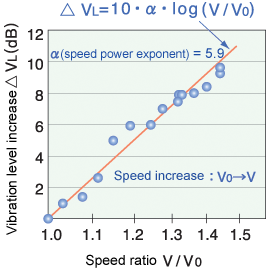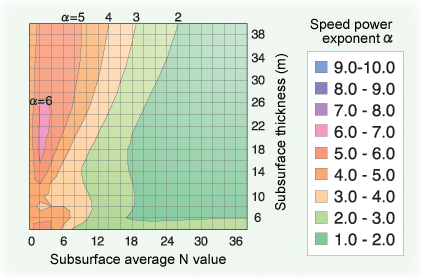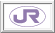3. Method to Evaluate the Influence of Speed Improvements on Ground Vibration

A method was devised to evaluate the influence of increasing Shinkansen speeds on wayside ground vibration. Ground vibration generally increases according to the exponential law of train speed, but the power exponent varies according to location. It has been assumed that the vibration level increases approximately according to the third power of velocity law for the Tokaido/Sanyo Shinkansen and approximately according to the second power of velocity law for the Tohoku/Joetsu Shinkansen. In recent years, however, some special cases have been found where the vibration level increases approximately according to the sixth power of velocity law (Fig.1).

The results of past speedup tests were therefore re-analyzed to examine the relationship between the speed power exponent and ground conditions in detail. The results clarified that the speed power exponent depends on the amplification characteristics of vibration of the subsurface layers. To evaluate this relationship quantitatively, a method was also devised to easily calculate the speed power exponent of the location's ground vibration from the average N value (an index indicating the hardness of the ground) and the thickness of the surface.

Calculation of the speed power exponent of the Shinkansen's ground vibration using this method showed that the speed power exponent roughly grew as the ground became thicker and weaker with a decreasing surface average N value (Fig.2).Fig.1 Example of increased vibration level with increasing speed Fig.2 Example of calculation of the speed power exponent using the subsurface average N value and thickness as parameters

HOME > Major Results of Research and Development in Fiscal 2003 > IV Harmonization with the EnvironmentRTRI HOME